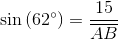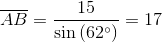## Example Questions

### Example Question #1 : How To Find The Length Of The Side Of A Parallelogram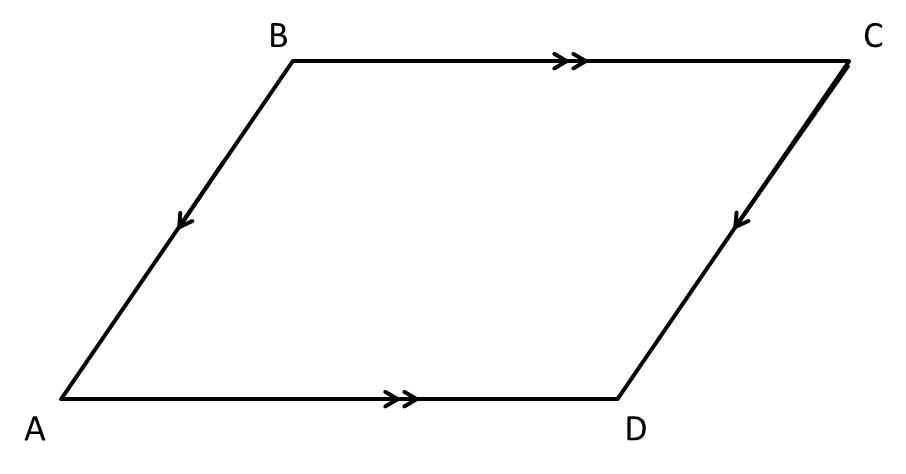In parallelogram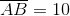and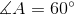. Find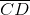.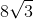There is insufficient information to solve the problem.Explanation:

In a parallelogram, opposite sides are congruent. Thus,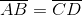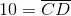### Example Question #2 : How To Find The Length Of The Side Of A ParallelogramIn parallelogram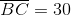and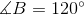. Find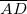.

There is insufficient information to solve the problem.Explanation:

In a parallelogram, opposite sides are congruent.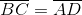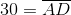### Example Question #3 : How To Find The Length Of The Side Of A Parallelogram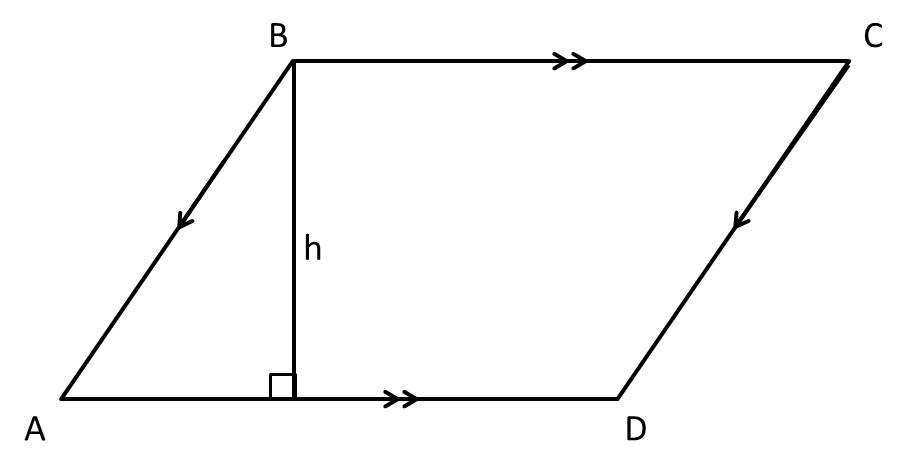Parallelogramhas an area of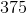. If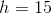, find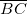.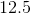There is insufficient information to solve the problem.Explanation:

The area of a parallelogram is given by: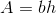In this problem, the height is given asand the area is. Bothandare bases.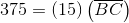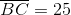### Example Question #4 : How To Find The Length Of The Side Of A Parallelogram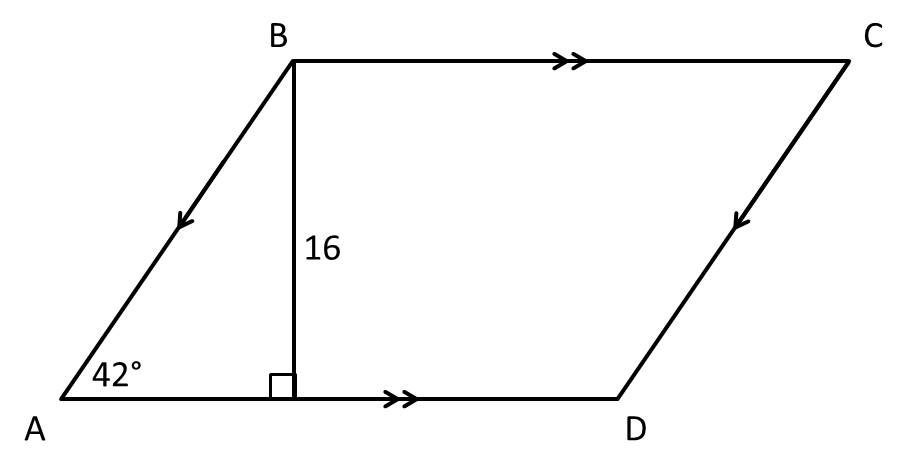is a parallelogram. Find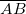.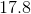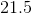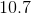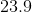There is insufficient information to solve the problem.Explanation:is the hypotenuse of the right triangle formed when we draw the height of the parallelogram. Because it is a right triangle, we can use SOH CAH TOA to solve for. With respect to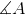, we know the opposite side of the triangle and we are looking for the hypotenuse. Thus, we can use the sine function to solve for.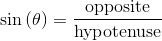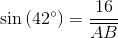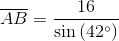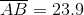### Example Question #5 : How To Find The Length Of The Side Of A Parallelogram

Find the length of the base of a parallelogram with a height ofand an area of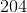.Explanation:

The formula for the area of a parallelogram is:By plugging in the given values, we get: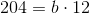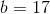### Example Question #6 : How To Find The Length Of The Side Of A Parallelogram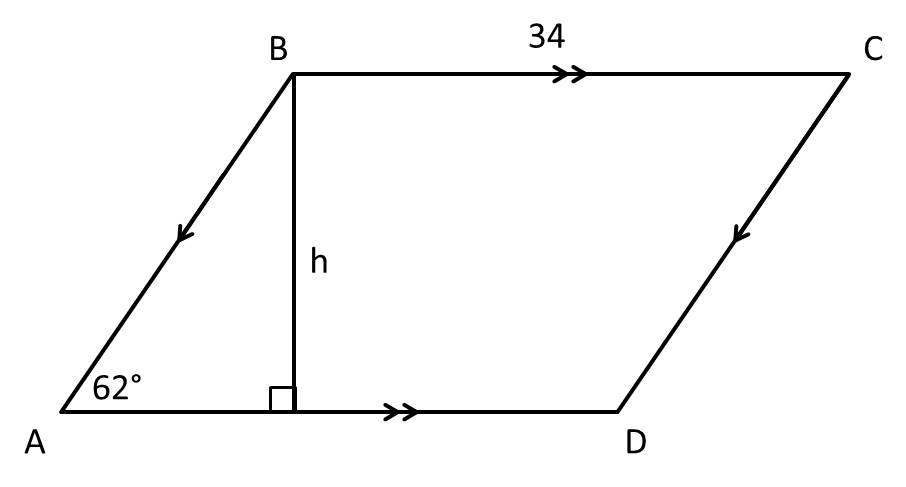is a parallelogram with an area of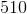. Find.There is insufficient information to solve the problem.Explanation:

In order to find, we must first find. The formula for the area of a parallelogram is:We are givenas the area andas the base.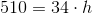Now, we can use trigonometry to solve for. With respect to, we know the opposite side of the right triangle and we are looking for the hypotenuse. Thus, we can use the sine function.